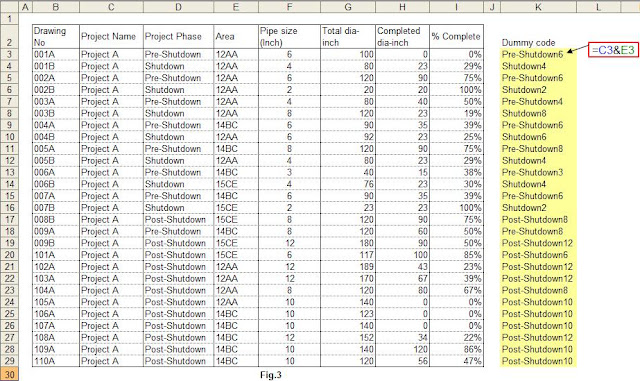## Pages

• Home
• Tutorials

### How to Use SUMIF Function for Progress Analysis

SUMIF function is used to sum up the required data which they match criteria. It is utilized to analyse the data in a database or spreadsheet. For example, the idea is that you want to get the total number of pipe diameter-inch produced in the week and less than 4” pipe size from a table or you may want to get the total number of goods sold in last month and more than profit margin and so on. The data can be analysed by one or more criteria using SUMIF function.
Suppose we already have a list of isometric drawings against the diameter-inch completed for a project as shown in Fig-1.Let assume that client has requested progress sheet that summarized by project phase and each pipe size.
Piping progress summary for this list can be prepared as below.
(1) Create a table that contain project phase and pipe size ( see Fig.2 ).(2) As we are analyzing the two criteria (project phase and pipe size) from the table ( Fig-1) , the dummy code should be created by joining the two criteria with "&" (An ampersand). See Fig-3.(3) When you type the formula, “=SUMIF” in the summary table (Fig-2), (range, criteria, [sum_range]) will be appeared. “Range” refers to the cell range that matches criteria. In this case cell range will be “Dummy code” column (see Fig-3) and select cell range (K3:K29) for 2" pipe size & Pre-Shutdown phase.
(4)Select the 1st criteria -Project phase such as Pre-shutdown, Shutdown and Post-shutdown, and 2nd criteria –Completed dia-inch. Use “&" between the 1st and 2nd criteria (C35&D34).
(5)As we are looking for the total number of completed dia-inch for each phase, “Completed dia-inch” column will be sum range (H3:H29).
(6) Add "\$" sign in that formula to copy. Copy the cells through the pipe size and project phase. Finally, you can find the total number of piping diameter for each size and project phase. See Fig.4

You may also want to analyse total completed diameter-inch by less than 6" pipe size and greater than 6". The return of pipe size (less than 6") is 167 dia-inch and for pipe size (greater than 6") is 753. Type the formula as shown in Fig-5.SUMIF function can be used with more than one criteria by combining the criteria codes with "&".